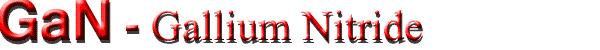## Band structure and carrier concentration

Basic Parameters
for Zinc Blende crystal structure
for Wurtzite crystal structure
Band structure
for Zinc Blende crystal structure
for Wurtzite crystal structure
Intrinsic carrier concentration
Effective Density of States in the Conduction and Valence Band
Temperature Dependences
Dependences on Hydrostatic Pressure
Band Discontinuities at Heterointerfaces
Effective Masses and Density of States
Donors and Acceptors
The Most Important Levels

### Basic Parameters

##### Zinc Blende crystal structure
 Remarks Referens Energy gaps, Eg 3.28 eV 0 K Bougrov et al. (2001) Energy gaps, Eg 3.2 eV 300 K Electron affinity 4.1 eV 300 K Conduction band Energy separation between Γ valley and X valleys EΓ 1.4 eV 300 K Bougrov et al. (2001) Energy separation between Γ valley and L valleys EL 1.6 ÷ 1.9 eV 300 K Effective conduction band density of states 1.2 x 1018 cm-3 300 K Valence band Energy of spin-orbital splitting Eso 0.02 eV 300 K Effective valence band density of states 4.1 x 1019 cm-3 300 K
##### Wurtzite crystal structure
 Remarks Referens Energy gaps, Eg 3.47 eV 0 K Bougrov et al. (2001) 3.39 eV 300 K Chow & Ghezzo (1996) Energy gaps, Eg,dir 3.503 (2) eV 1.6 K; photoluminescence, from excitonic gap adding the exciton binding energy Monemar (1974) 3.4751(5) eV 1.6 K; A-exciton (transition from Γ9v) 3.4815(10) eV 1.6 K; B-exciton (transition from upper Γ7v) 3.493 (5) eV 1.6 K; C-exciton (transition from lower Γ7v) 3.44 eV 300K; temperature dependence below 295 K given by: Eg(T) - Eg(0) = - 5.08 x 10-4 T2/(996 - T), (T in K) . see also Figure "Band gap energy and exciton energies vs. temperature" Madelung (1991) Electron affinity 4.1 eV 300 K Bougrov et al. (2001)
 Remarks Referens Conduction band Energy separation between  Γ valley and M-L valleys 1.1 ÷ 1.9 eV 300 K Bougrov et al. (2001) Energy separation between  M-L-valleys degeneracy 6 eV 300 K Energy separation between   Γ valley and A valleys 1.3 ÷ 2.1 eV 300 K Energy separation between   A-valley degeneracy 1 eV 300 K also The energy separations between the Γ9 state and the two Γ 7 states can be calculated from the energy separations of the A-, B-, C-excitons. Madelung (1991) Effective conduction band density of states 2.3 x 1018 cm-3 300 K Valence band Energy of spin-orbital splitting Eso 0.008 eV 300 K Bougrov et al. (2001) Energy of spin-orbital splitting Eso 11(+5,-2) meV 300 K; calculated from the values of energy gap Eg,dir (given above) Dingle & Ilegems (1971) Energy of crystal-field splitting Ecr 0.04 eV 300 K Bougrov et al. (2001) Energy of crystal-field splitting Ecr 22(2) meV 300 K; calculated from the values of energy gap Eg,dir (given above) Dingle & Ilegems (1971) Effective valence band density of states 4.6 x 1019 cm-3 300 K

### Band structure for Zinc Blende GaN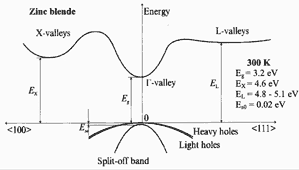Band structure of zinc blende(cubic) GaN. Important minima of the conduction band and maxima of the valence band. 300K; Eg =3.2 eV eV; EX= 4.6 eV; EL= 4.8-5.1 eV; Eso = 0.02 eV For details see Suzuki, Uenoyama & Yanase (1995) .Brillouin zone of the face centered cubic lattice, the Bravais lattice of the diamond and zincblende structures.

### Band structure for Wurtzite GaN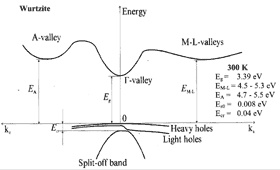GaN, Wurtzite. Band structure. Important minima of the conduction band and maxima of the valence band. Valence band 3 splitted bands. This splitting results from spin-orbit interaction and from crystal symmetry. 300K; Eg =3.39 eV eV; EM-L= 4.5-5.3 eV; EA= 4.7-5.5 eV; Eso = 0.008 eV; Ecr = 0.04 eV For details see Suzuki, Uenoyama & Yanase (1995) .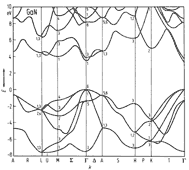GaN, Wurtzite. Band structure calculated with an empirical pseudopotential method The band structure differs only slightly from other spin-neglecting calculations. Introduction of spin-orbit interaction leads to a splitting of the uppermost valence band at Γ from Γ1 + Γ6 , into Γ9 + Γ7 + Γ7 . The energy differences between these terms can be described by two parameters - the spin-orbit splitting energy Eso (Δso) and the crystal field splitting energy Ecr(Δcr). Also see Basic Parameters. Bloom et al.(1974)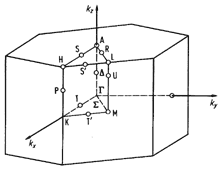Brillouin zone of the hexagonal lattice.

### Temperature Dependences

The energy gap versus temperature:
 Eg = Eg(0) - 7.7·10-4x T2/(T + 600) (eV) Bougrov et al. (2001 Eg(0) = 3.47 eV (wurtzite)       Eg(0) = 3.28 eV (zinc blende) Eg = Eg(0) - 9.39 x 10-4 x T2/(T + 772) (eV) Guo & Yoshida (1994) Teisseyre al. (1994) Eg (0 K) = 3.427 eV
where T is temperature in degrees K
 Varshni expression: Eg = Eg(0) - 9.39·10-4x T2/(T + 772) (eV) Guo & Yoshida (1994), Teisseyre al. (1994) where Eg(0) = 3.427 eV and  T is temperature in degrees K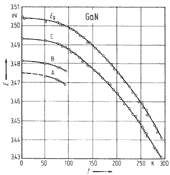GaN, Wurtzite. Band gap energy and exciton energies vs. temperature Monemar (1974) Temperature dependence below 295 K given by: Eg(T) - Eg(0) = - 5.08 x 10-4 T2/(996 - T), (T in K). Eg(300K) =3.44 eV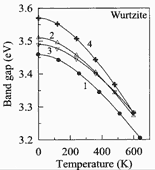GaN, Wurtzite. Band gap energy versus temperature. GaN samples were grown on different substrates using different techniques. Experimental data are taken from four different works. Bougrov et al. (2001)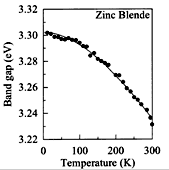GaN, Zinc Blende(cubic). The Band gap energy versus temperature. GaN films were grown on MgO (1x1) substrates. Ramirez-Flores et al. (1994)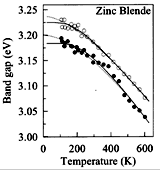GaN, Zinc Blende(cubic). The Band gap energy versus temperature. GaN films were grown on Si (100) substrates. The dependences were extracted from pseudodielectric-function spectrum using two different theoretical models Petalas et al. (1995)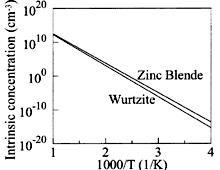GaN, Wurtzite & Zinc Blende. The intrinsic carrier concentration vs. temperature. Bougrov et al. (2001)

### Intrinsic carrier concentration:

ni = (Nc·Nv)1/2exp(-Eg/(2kBT))

### Effective density of states in the conduction band: Nc

##### Wurtzite
Nc ~= 4.82 x 1015 · (mΓ/m0)3/2T3/2 (cm-3) ~= 4.3 x 1014 x T3/2 (cm-3)
##### Zinc Blende
Nc ~= 4.82 x 1015 · (mΓ/m0)3/2T3/2 (cm-3) ~= 2.3 x 1014 x T3/2 (cm-3)

### Effective density of states in the valence band: Nv

##### Wurtzite
Nv = 8.9 x 1015 x T3/2 (cm-3)
##### Zinc Blende BN
Nv = 8.0 x 1015 x T3/2 (cm-3)

### Dependence on Hydrostatic Pressure

##### Wurtzite GaN
Eg = Eg(0) + 4.2 x 10-3P-1.8x 10-5P2        (eV)�
where P is pressure in kbar. Morkoc et al. (1994), Akasaki & Amano (1994a).

### Band Discontinuities at Heterointerfaces

##### Wurtzite GaN
 AIN/GaN(0001) Referens Conduction band discontinuity ΔEc = 2.0 eV Martin et al. (1996) Valence band discontinuity ΔEv = 0.7 eV InN/GaN Conduction band discontinuity ΔEc = 0.43 eV Martin et al. (1996) Valence band discontinuity ΔEv = 1.0 eV
##### Zinc Blende BN (cubic)
 GaAs/GaN Referens Valence band discontinuity ΔEv = 1.84 eV Ding et al. (1997)

### Effective Masses and Density of States:

#### Electrons

For wurtzite crystal structure the surfaces of equal energy in Γ valley should be ellipsoids, but effective masses in z direction and perpendicular directions are estimated to be approximately the same:
 Effective Masses for Zinc Blende GaN Remarks Referens Effective electron mass  me 0.13 mo 300 K Bougrov et al. (2001) Effective Masses for Wurtzite GaN Effective electron mass  me 0.20 mo 300 K Bougrov et al. (2001) 0.27 (6) mo 300K; Faraday rotation Rheinlander & Neumann (1974) Effective electron mass me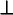0.20(2) mo 300K; fit of reflectance spectrum Bloom et al.(1973) Effective electron mass me|| 0.20(6) mo

#### Holes

For zinc blende crystals, only calculated data are available.
 Effective Masses for Zinc Blende GaN Remarks Referens Effective mass of density of state mv mv = 1.4 mo Effective hole masses (heavy) mhh mhh = 1.3 mo m = 0.8 mo m = 1.7 mo 300 K Leszczynski et al. (1996), Fan et al. (1996) Effective hole masses (light) mlp mlh = 0.19mo m = 0.21 mo m = 0.18 mo 300 K Effective hole masses (split-off band) ms msh = 0.33 mo m = 0.33 mo m = 0.33 mo 300 K

Experimental data for wurtzite crystals give the value of hole effective mass about l.0 m0. This value is less than the calculated one.
 Effective Masses for Wurtzite GaN Remarks Referens Effective mass of density of state mv 1.5 m0 Leszczynski et al. (1996), Fan et al. (1996) Effective hole masses 0.8 (2) mo 300 K, experimental Pankove et al.(1975) Effective hole masses (heavy) mhh mhh = 1.4 mo mhhz = 1.1 mo mhh= 1.6 mo 300 K; calculated values Leszczynski et al. (1996), Fan et al. (1996) Effective hole masses (light) mlp 0.259 mo 300 K; calculated values Effective hole masses (light) mlh = 0.3 mo mlhz = 1.1 mo mlh= 0.15 mo 300 K; calculated values Leszczynski et al. (1996), Fan et al. (1996) Effective hole masses (split-off band) ms msh = 0.6 mo mshz = 0.15 mo msh= 1.1 mo 300 K; calculated values Leszczynski et al. (1996), Fan et al. (1996)

### Donors and Acceptors

##### Zinc Blende (cubic) GaN
For cubic GaN, only calculated data are available. [see Neugebauer & Van de Walle (1994), Boguslawski et al. (1995), Mattila et al. (1996), Boguslawski & Bernholc (1996), Gorczyca et al. (1997)].
##### Wurtzite GaN
 Ionization energies of Shallow Donors Si 0.12-0.02 eV Bougrov et al. (2001) Native defect level VN 0.03 eV Ionization energies of Shallow Acceptor Mg 0.14-0.21 eV Strite & Morkoc (1992), Akasaki & Amano (1994b) Zn 0.21 eV Native defect level VGa 0.14 eV

### The Most Important Levels

##### Wurtzite GaN
Donors, lonization Energy (Et - Ec)
 Ipurity or Defect Ga Site N Site Referens Si 0.12-0.02 eV Bougrov et al. (2001) VN (vacancy) 0.03; 0.1 eV C 0.11-0.14 eV Mg 0.26; 0.6 eV
Acceptors, lonization Energy (Ev - Et)
 Ipurity or Defect Ga Site N Site Referens VGa (vacancy) 0.14 Bougrov et al. (2001) Mg 0.14-0.21 eV Si 0.19 eV Zn 0.21-0.34 eV Hg 0.41 eV Cd 0.55 eV Be 0.7 eV Li 0.75 eV C 0.89 eV Ga 0.59-1.09 eV